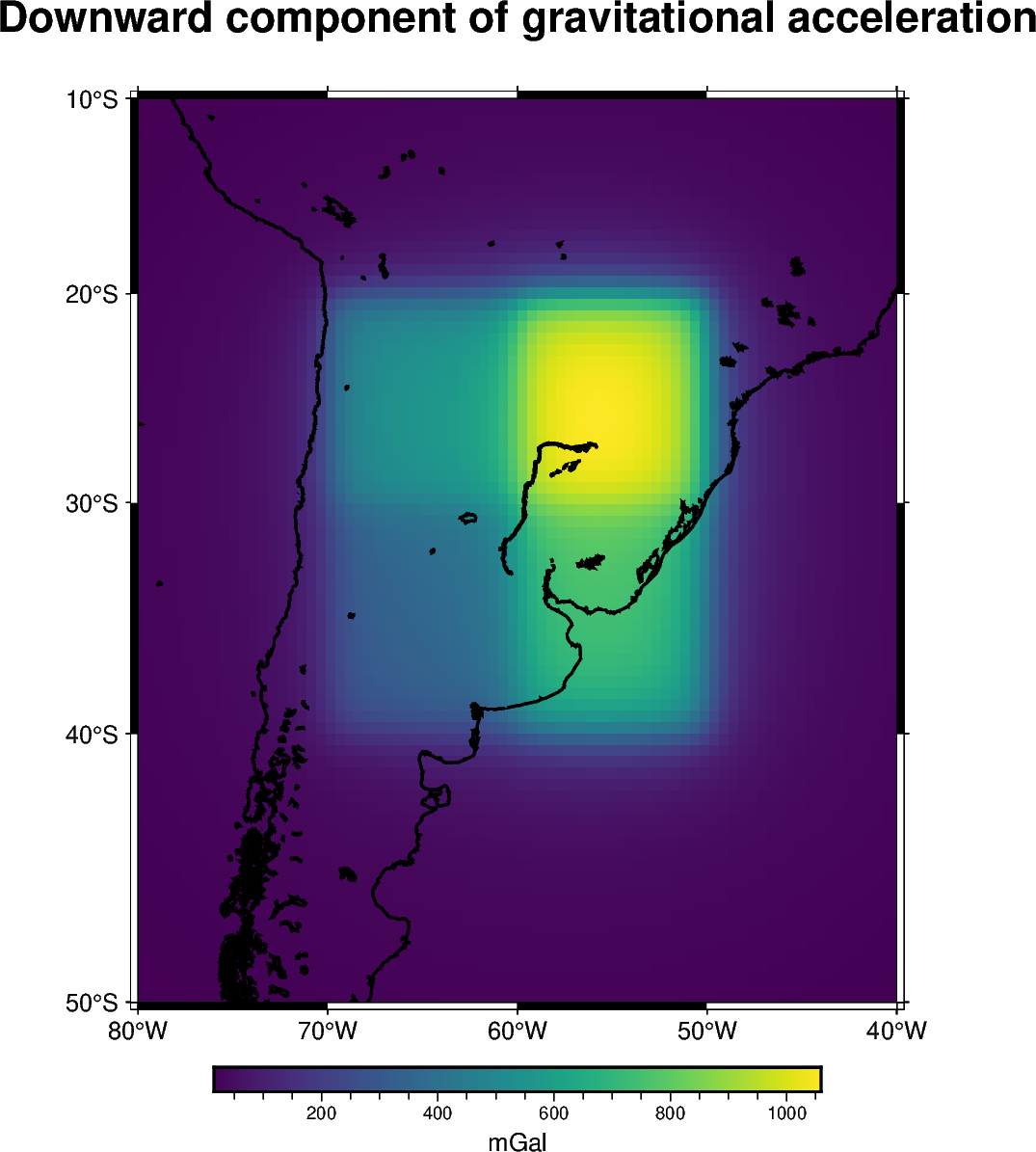# Tesseroids with variable density¶

The `harmonica.tesseroid_gravity` is capable of computing the gravitational effects of tesseroids whose density is defined through a continuous function of the radial coordinate. This is achieved by the application of the method introduced in [Soler2021].

To do so we need to define a regular Python function for the density, which should have a single argument (the `radius` coordinate) and return the density of the tesseroids at that radial coordinate. In addition, we need to decorate the density function with `numba.jit(nopython=True)` or `numba.njit` for short.

On this example we will show how we can compute the gravitational effect of four tesseroids whose densities are given by a custom linear `density` function.Out:

```[[15.64931026 15.84632554 16.04629019 ... 17.73106017 17.50445005
17.28024265]
[15.93321711 16.14345748 16.35722954 ... 18.16928493 17.9230556
17.68001566]
[16.2246854  16.4491229  16.6777669  ... 18.62722145 18.3593706
18.09566506]
...
[15.52398623 15.81724318 16.12003228 ... 18.50055111 18.12075672
17.75302149]
[15.24656009 15.52448978 15.8108793  ... 18.06017674 17.705425
17.36104098]
[14.97689905 15.24046063 15.51153133 ... 17.6376896  17.30600261
16.98321625]]
```

```import boule as bl
import cartopy.crs as ccrs
import matplotlib.pyplot as plt
import verde as vd
from numba import njit

import harmonica as hm

# Use the WGS84 ellipsoid to obtain the mean Earth radius which we'll use to
# reference the tesseroid
ellipsoid = bl.WGS84

# Define tesseroid with top surface at the mean Earth radius, a thickness of
# 10km and a linear density function
tesseroids = (
)

# Define a linear density function. We should use the jit decorator so Numba
# can run the forward model efficiently.

@njit
"""Linear density function"""
density_top = 2670
density_bottom = 3000
slope = (density_top - density_bottom) / (top - bottom)
return slope * (radius - bottom) + density_bottom

# Define computation points on a regular grid at 100km above the mean Earth
coordinates = vd.grid_coordinates(
region=[-80, -40, -50, -10],
shape=(80, 80),
)

# Compute the radial component of the acceleration
gravity = hm.tesseroid_gravity(coordinates, tesseroids, density, field="g_z")
print(gravity)

# Plot the gravitational field
fig = plt.figure(figsize=(8, 9))
ax = plt.axes(projection=ccrs.Orthographic(central_longitude=-60))
img = ax.pcolormesh(*coordinates[:2], gravity, transform=ccrs.PlateCarree())
plt.colorbar(img, ax=ax, pad=0, aspect=50, orientation="horizontal", label="mGal")
ax.coastlines()
ax.set_title("Downward component of gravitational acceleration")
plt.show()
```

Total running time of the script: ( 0 minutes 2.801 seconds)

Gallery generated by Sphinx-Gallery